Holt.Blue

Cryptography: A Less-Than-Complete Introduction in Five Acts

Benjamin V. Holt

Southwestern STEM Colloquium

November 8th, 2019

Cryptography is an ancient and modern practice.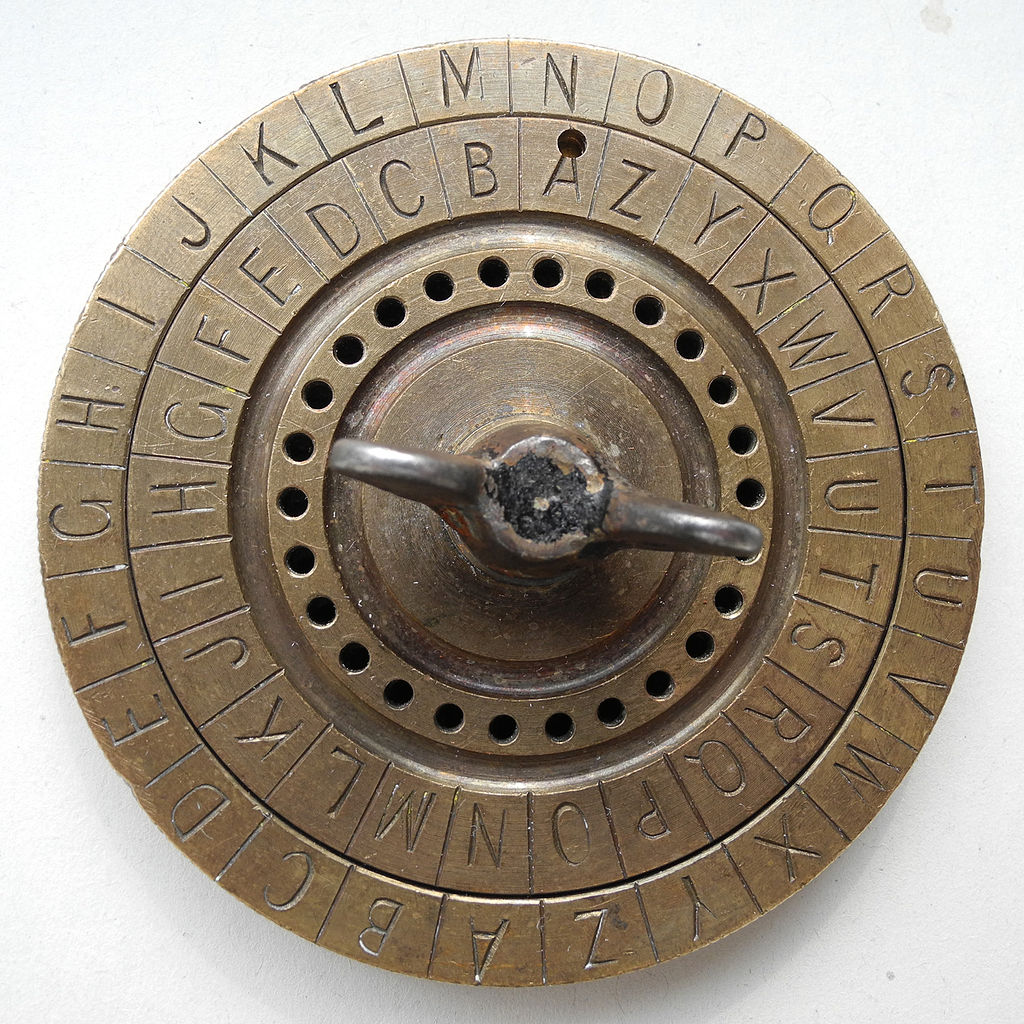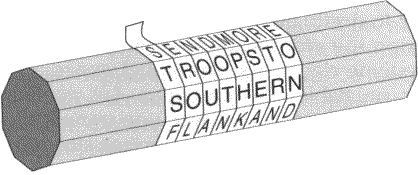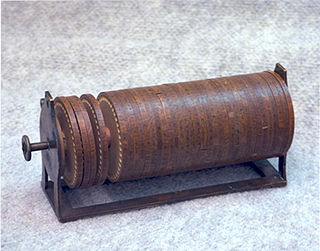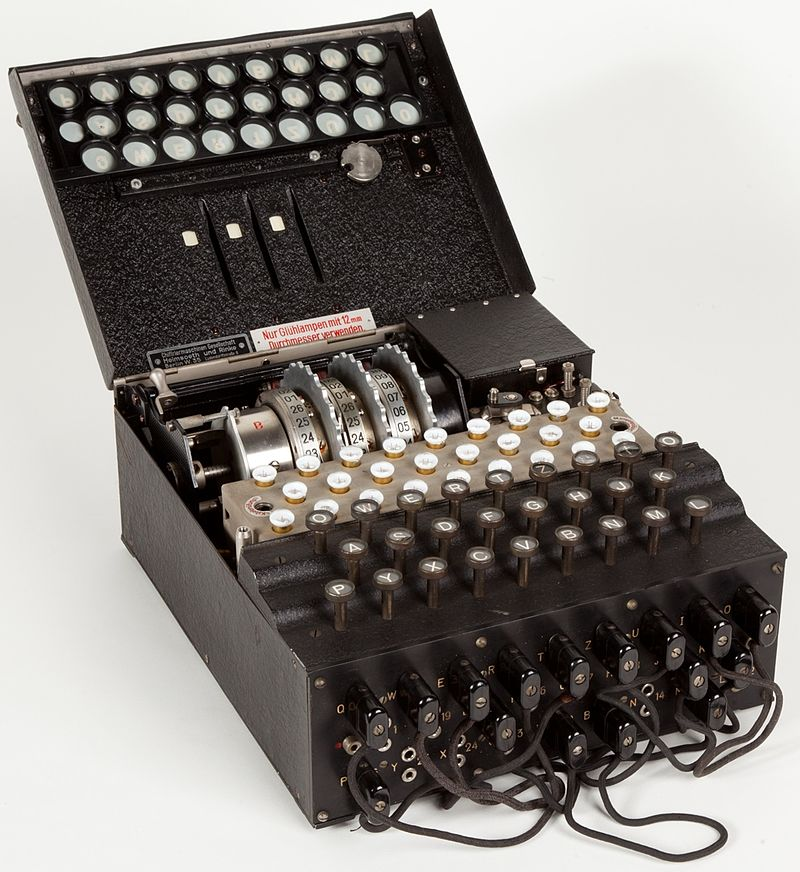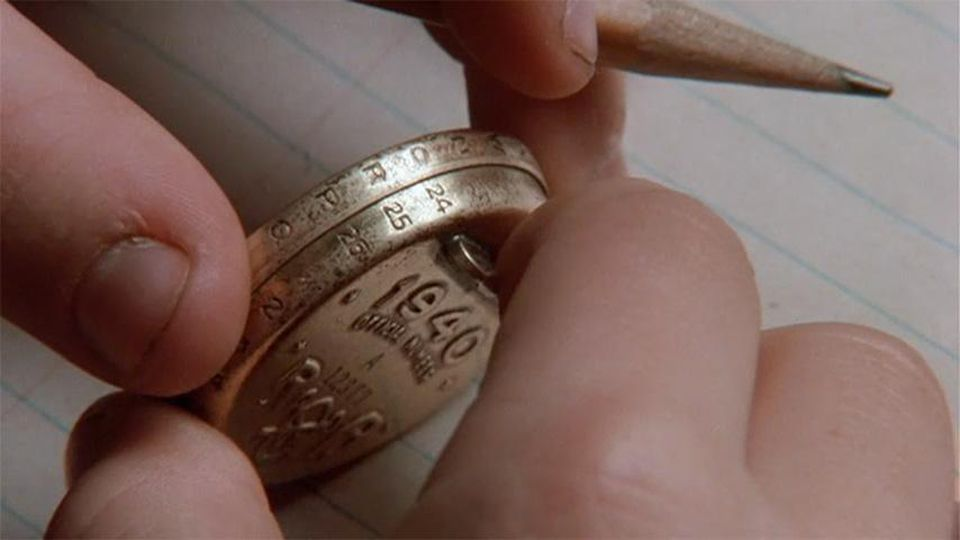Act I

A Whirlwind Tour of Modular Arithmetic

Modular Arithmetic

Modular arithmetic is remainder arithmetic.

Two numbers are "equal" if they have the same remainder when dividing by a fixed number called the modulus.

For example, $19$ and $10$ have the same remainder when we divide both by $9.$

We may write the above as $19 \equiv 10 \equiv 1 \,\,(\mbox{mod } 9).$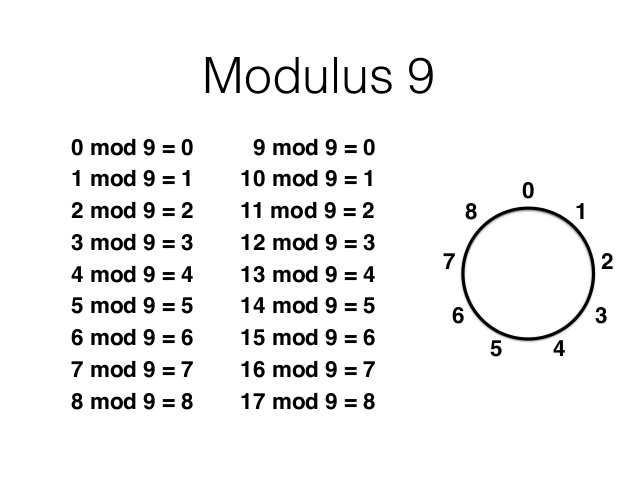Modular Arithmetic

If you can answer the following question, then you are already familiar with one example of modular arithmetic:

It's 11 am. What time will it be in 4 hours?

Modular Arithmetic

Yes! Colloquium time!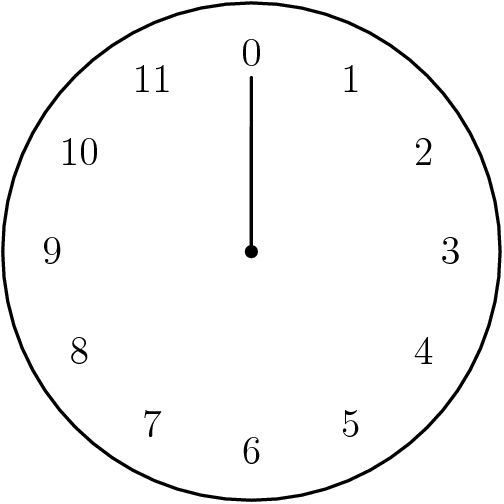We just performed arithmetic modulo $12$: $$11+4 \equiv 15 \equiv 3 \,\,(\mbox{mod } 12).$$

Modular ArithmeticModular Arithmetic: Examples.
 $9+7 \equiv \,\,\,\,(\mbox{mod } 12)$ $9 \cdot 7 \equiv \,\,\,\,(\mbox{mod } 12)$$8+3 \equiv \,\,\,\,(\mbox{mod } 9)$ $8 \cdot 3 \equiv \,\,\,\,(\mbox{mod } 9)$Act II

A Brief Encounter with Private-Key Cryptography

Big Fact: Modular arithmetic allows us to perform mathematical operations on the alphabet!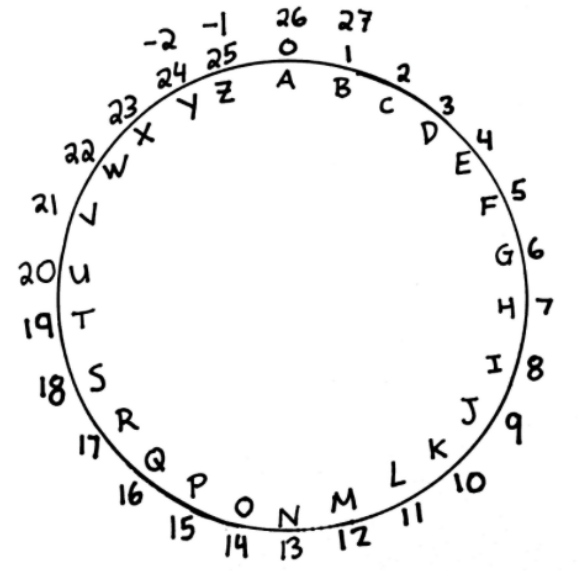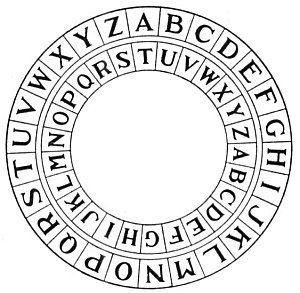For example, $$\begin{array}{c} \mbox{H} = 7 \rightarrow H+T=7+19 \equiv 0 = A\\ \end{array}$$

Big Fact: Modular arithmetic allows us to perform mathematical operations on the alphabet!$$\begin{array}{lcc} \mbox{H} = 7 &\rightarrow &\mbox{H}+\mbox{T}=7+19 \equiv 0 = \mbox{A}\\ \mbox{E} = 4 &\rightarrow& \mbox{E}+\mbox{T}=4+19 \equiv 23 = \mbox{X}\\ \mbox{L} = 11 &\rightarrow& \mbox{L}+\mbox{T}=11+19 \equiv 4 = \mbox{E}\\ \mbox{P} = 15 &\rightarrow &\mbox{P}+\mbox{T}=15+19 \equiv 8 = \mbox{I}\\ \end{array}$$

The Caesar Cipher

To encrypt a letter $p$, shift it to the left or right by some number of spaces $e$ using our decoder pin:Or simply use modular arithmetic: $$p \rightarrow p+e \equiv c \,\,(\mbox{mod } 26)$$ The value $e$ is our encryption key, and for the above $e=T=19.$ This is what gave us the encrypted message $$\mbox{HELP}\rightarrow \mbox{AXEI}.$$

Big Question: Suppose we received the encrypted message $\mbox{AXEI}.$ How would we decrypt it?

The Caesar Cipher

To decrypt a letter $c$, shift it to the left or right by some number of spaces $d$ in the reverse direction of the encryption key $e$:Or simply use modular arithmetic: $$c \rightarrow c-e \equiv c+d \equiv p \,\,(\mbox{mod } 26)$$ Our decryption key for the above example is $$d \equiv -e \equiv -19 \equiv 7 =\mbox{H} \,\,(\mbox{mod } 26).$$

The Caesar Cipher

To decrypt a letter $c$, shift it to the left or right by some number of spaces $d$ in the reverse direction of the encryption key $e$:$$\begin{array}{lcc} \mbox{A} = 0 &\rightarrow& \mbox{A}+\mbox{H}=0+7 \equiv 7 = \mbox{H}\\ \mbox{X} = 23 &\rightarrow& \mbox{X}+\mbox{H}=23+7 \equiv 4 = \mbox{E}\\ \mbox{E} = 4 &\rightarrow& \mbox{E}+\mbox{H}=4+7 \equiv 11 = \mbox{L}\\ \mbox{I} = 8 &\rightarrow& \mbox{I}+\mbox{H}=8+7 \equiv 15 = \mbox{P}\\ \end{array}$$

Other Schemes: Affine Cipher $$p \rightarrow a \cdot p+e \equiv c \,\,(\mbox{mod } 26)$$ where our key is an ordered pair $(a,e)$ where $a$ and $26$ have no common prime factors.

Example: With $(a,e)=(3,19),$ $$\begin{array}{lcc} \mbox{H} = 7 &\rightarrow & 3 \cdot 7+19 \equiv 14 = \mbox{O}\\ \mbox{E} = 4 &\rightarrow& 3 \cdot 4+19 \equiv 5 = \mbox{F}\\ \mbox{L} = 11 &\rightarrow& 3 \cdot 11+19 \equiv 0 = \mbox{A}\\ \mbox{P} = 15 &\rightarrow & 3 \cdot 15+19 \equiv 12 = \mbox{M}\\ \end{array}$$

Big Problem

The Caesar and Affine Ciphers share a key weakness. Can you spot it?

Frequency Analysis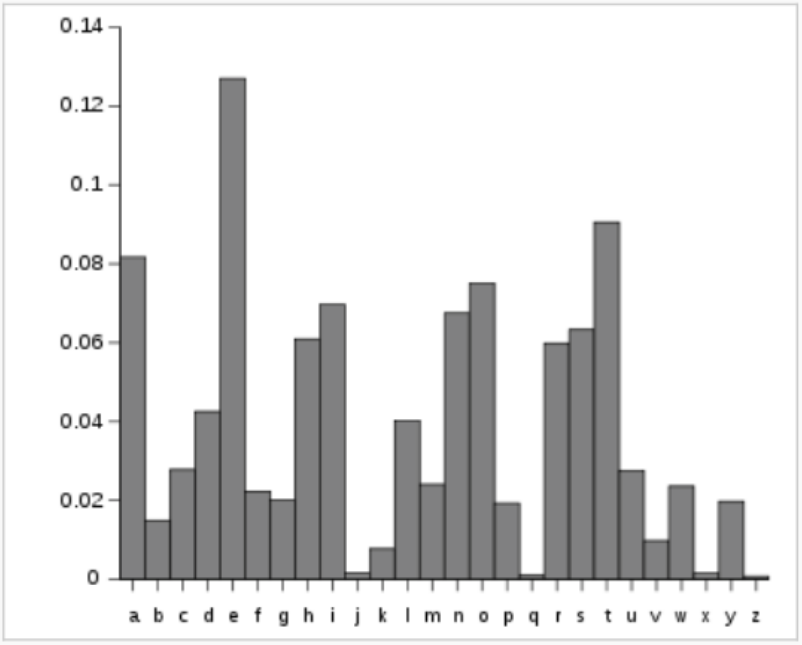Other Schemes: Vigenère Cipher $$\left[ \begin{array}{c} p_1 \\ p_2 \\ \vdots\\ p_n \end{array} \right]\rightarrow \left[ \begin{array}{c} p_1+e_1 \\ p_2+e_2 \\ \vdots\\ p_n+e_n \end{array} \right] \equiv \left[ \begin{array}{c} c_1 \\ c_2\\ \vdots\\ c_n \end{array} \right] \,\,(\mbox{mod } 26)$$ Where our encryption key is an $n$-letter "word" $(e_1,e_2,\ldots, e_n).$

Example: With key word $(e_1,e_2,e_3,e_4)=(\mbox{S},\mbox{O},\mbox{O},\mbox{N})=(18,14,14,13),$ $$\left[ \begin{array}{c} \mbox{H} \\ \mbox{E} \\ \mbox{L} \\ \mbox{P} \end{array} \right] = \left[ \begin{array}{c} 7 \\ 4 \\ 11 \\ 15 \end{array} \right] \rightarrow \left[ \begin{array}{c} \mbox{H}+\mbox{S} \\ \mbox{E}+\mbox{O} \\ \mbox{L}+\mbox{O}\\ \mbox{P}+\mbox{N} \end{array} \right] = \left[ \begin{array}{c} 7+18 \\ 4+14 \\ 11+14\\ 15+13 \end{array} \right] \equiv \left[ \begin{array}{c} 25 \\ 18\\ 25\\ 2 \end{array} \right] = \left[ \begin{array}{c} \mbox{Z} \\ \mbox{S}\\ \mbox{Z}\\ \mbox{C} \end{array} \right] \,\,(\mbox{mod } 26)$$

Bonus Example: The key word doesn't necessarily need to be the same length as the message.

With key word $(e_1,e_2)=(\mbox{M},\mbox{E})=(12,4),$ we can encrypt in "blocks" of length $2$: $$\left[ \begin{array}{c} \mbox{H} \\ \mbox{E} \\ \mbox{L} \\ \mbox{P} \end{array} \right] = \left[ \begin{array}{c} 7 \\ 4 \\ 11 \\ 15 \end{array} \right] \rightarrow \left[ \begin{array}{c} \mbox{H}+\mbox{M} \\ \mbox{E}+\mbox{E} \\ \mbox{L}+\mbox{M}\\ \mbox{P}+\mbox{E} \end{array} \right] = \left[ \begin{array}{c} 7+12 \\ 4+4 \\ 11+12\\ 15+4 \end{array} \right] \equiv \left[ \begin{array}{c} 19 \\ 8\\ 23\\ 19 \end{array} \right] = \left[ \begin{array}{c} \mbox{T} \\ \mbox{I}\\ \mbox{X}\\ \mbox{T} \end{array} \right] \,\,(\mbox{mod } 26)$$

Other Schemes: Hill Cipher $$\left[ \begin{array}{c} p_1 \\ p_2 \\ \vdots\\ p_n \end{array} \right]\rightarrow \left[ \begin{array}{cccc} e_{11} & e_{12} & \cdots & e_{1n}\\ e_{21} & e_{22} & \cdots & e_{2n}\\ \vdots & \vdots & \ddots & \vdots\\ e_{n1} & e_{n2} & \cdots & e_{nn}\\ \end{array} \right] \left[ \begin{array}{c} p_1 \\ p_2\\ \vdots\\ p_n \end{array} \right] \equiv \left[ \begin{array}{c} c_1 \\ c_2\\ \vdots\\ c_n \end{array} \right] \,\,(\mbox{mod } 26)$$ The encryption key is the above $n \times n$ matrix which we assume has a determinant of $1$ modulo $26.$

Example: In blocks of $2,$ our message becomes $\,\,\,\,\mbox{H}\mbox{E}\,\,\,\,\mbox{L}\mbox{P}$ $$\left[ \begin{array}{c} \mbox{H} \\ \mbox{E} \\ \end{array} \right] = \left[ \begin{array}{c} 7 \\ 4 \\ \end{array} \right] \rightarrow \left[ \begin{array}{cc} 5 & 3\\ 1 & 6\\ \end{array} \right] \left[ \begin{array}{c} 7 \\ 4\\ \end{array} \right] = \left[ \begin{array}{c} 5 \cdot 7+3 \cdot 4 \\ 1 \cdot 7+6 \cdot 4\\ \end{array} \right] \equiv \left[ \begin{array}{c} 21 \\ 5\\ \end{array} \right] = \left[ \begin{array}{c} \mbox{V} \\ \mbox{F} \\ \end{array} \right] \,\,(\mbox{mod } 26)$$ $$\left[ \begin{array}{c} \mbox{L} \\ \mbox{P} \\ \end{array} \right] = \left[ \begin{array}{c} 11 \\ 15 \\ \end{array} \right] \rightarrow \left[ \begin{array}{cc} 5 & 3\\ 1 & 6\\ \end{array} \right] \left[ \begin{array}{c} 11 \\ 15\\ \end{array} \right] = \left[ \begin{array}{c} 5 \cdot 11+3 \cdot 15 \\ 1 \cdot 11+6 \cdot 15\\ \end{array} \right] \equiv \left[ \begin{array}{c} 22 \\ 23\\ \end{array} \right] = \left[ \begin{array}{c} \mbox{X} \\ \mbox{Y} \\ \end{array} \right] \,\,(\mbox{mod } 26)$$ The encrypted message is then $\mbox{VFXY}.$

Bonus Example: To decrypt the message $\mbox{VFXY}$ we again break our message into blocks of $2$: $\,\,\,\,\mbox{V}\mbox{F}\,\,\,\,\mbox{X}\mbox{Y}.$ Then multiply by the modulo-$26$ inverse of the encrypting matrix. $$\left[ \begin{array}{c} \mbox{V} \\ \mbox{F} \\ \end{array} \right] = \left[ \begin{array}{c} 21 \\ 5 \\ \end{array} \right] \rightarrow \left[ \begin{array}{cc} 6 & 23\\ 25 & 5\\ \end{array} \right] \left[ \begin{array}{c} 21 \\ 5\\ \end{array} \right] = \left[ \begin{array}{c} 6 \cdot 21+23 \cdot 5 \\ 25 \cdot 21+5 \cdot 5\\ \end{array} \right] \equiv \left[ \begin{array}{c} 7 \\ 4\\ \end{array} \right] = \left[ \begin{array}{c} \mbox{H} \\ \mbox{E} \\ \end{array} \right] \,\,(\mbox{mod } 26)$$ $$\left[ \begin{array}{c} \mbox{X} \\ \mbox{Y} \\ \end{array} \right] = \left[ \begin{array}{c} 22 \\ 23 \\ \end{array} \right] \rightarrow \left[ \begin{array}{cc} 6 & 23\\ 25 & 5\\ \end{array} \right] \left[ \begin{array}{c} 22 \\ 23\\ \end{array} \right] = \left[ \begin{array}{c} 6 \cdot 22+23 \cdot 23 \\ 25 \cdot 22+5 \cdot 23\\ \end{array} \right] \equiv \left[ \begin{array}{c} 11 \\ 25\\ \end{array} \right] = \left[ \begin{array}{c} \mbox{L} \\ \mbox{P} \\ \end{array} \right] \,\,(\mbox{mod } 26)$$

Fact: Among their many limitations, all of the above schemes have a particular drawback which makes them unsuitable for publicly exchanging information in our modern age...

...both the sender and receiver of a message must have the encryption/decryption key.

All of the encryption/decryption schemes we have considered so far are examples of private-key cryptosystems.

Observation: knowing how a sender encrypted a message tells us how to decrypt it.

Act II Cliffhanger

Is there a scheme where knowing how a message was encrypted doesn't tell us how to decrypt it?

Act III

Modular Arithmetic Redux: Fermat's Little Theorem

Fermat's Little Theorem

If a prime $p$ does not divide $a$, then $$a^{p-1} \equiv 1 \,\,(\mbox{mod } p).$$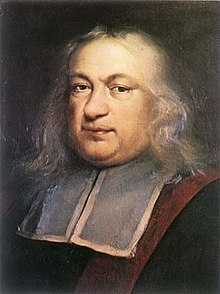Examples

$$4^{2} \equiv \,\,\,\,(\mbox{mod } 3).$$ $$8^{2} \equiv \,\,\,\,(\mbox{mod } 3).$$ $$2^{4} \equiv \,\,\,\,(\mbox{mod } 5).$$ $$3^{4} \equiv \,\,\,\,(\mbox{mod } 5).$$ $$2^{6} \equiv \,\,\,\,(\mbox{mod } 7).$$ $$2^{10} \equiv \,\,\,\,(\mbox{mod } 11).$$

A Slight Generalization

If primes $p$ and $q$ do not divide $a$, then $$a^{(p-1)(q-1)} \equiv 1 \,\,(\mbox{mod } pq).$$

If the natural numbers $n$ and $a$ have no prime factors in common, then $$a^{\varphi(n)} \equiv 1 \,\,(\mbox{mod } n).$$ The function $\varphi(n)$ is Euler's Totient function which is the number of natural numbers less than $n$ which have no prime factors in common with $n.$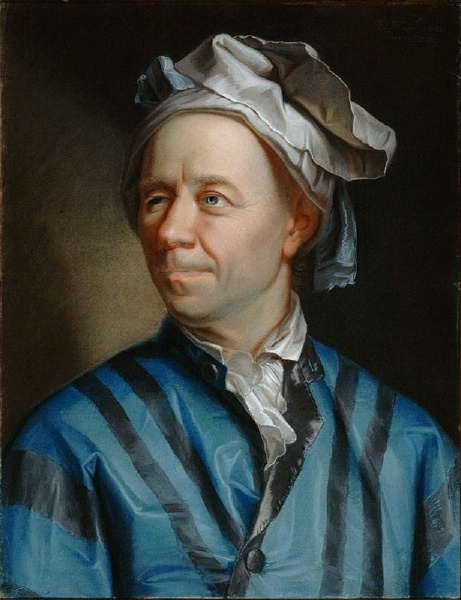If primes $p$ and $q$ do not divide $a$, and $e$ and $d$ are two numbers such that $${ed} \equiv 1 \,\,(\mbox{mod } (p-1)(q-1)),$$ then $$a^{ed} \equiv a \,\,(\mbox{mod } pq).$$

Act IV

The World's First Public-Key Cryptographic Scheme: RSA

The Situash: Bob would like to receive a secret message from Alice.

Moreover, Bob would like to be able to make the method of encryption public so that anyone could send him an encrypted message, but only Bob can decrypt the message.

Question: Is this even possible?

RSA System Basics

Step 1: Bob finds two large primes $p$ and $q$ and computes their product $n=pq.$

Step 2: Bob then finds two numbers $e$ and $d$ such that $${ed} \equiv 1 \,\,(\mbox{mod } (p-1)(q-1)).$$ Step 3: Bob publishes the key $(e,n)$ where $e$ is an encryption exponent and $n.$

Step 4: Alice encrypts the message $a$ by computing $a^e \,\,(\mbox{mod } n)$, and sends this to Bob.

Step 5: Bob takes the remainder of $a^{e}$ upon division by $n$ and raises it to the power of his decryption exponent $d$ so that $$(a^e)^d \equiv a^{ed} \equiv a \,\,(\mbox{mod } n).$$

Example

Step 1: Bob chooses two "large" primes $p=53$ and $q=59$ and computes $n=pq=3127.$

Step 2: Bob then finds two numbers $e$ and $d$ such that $ed \equiv 1 \,\,(\mbox{mod } 3016).$ For this example, $e=7$ and $d=431$ will do the trick.

Step 3: Bob publishes the encryption key $(7,3127).$

Step 4: Alice encrypts the message $\mbox{HELP}$ in blocks of 2: $0704^7 \equiv 254 \,\,(\mbox{mod } 3127)$ and $1115^7 \equiv 1612 \,\,(\mbox{mod } 3127)$ and sends the message $0254\,\,1612$ to Bob.

Step 5: Bob uses his decrypting exponent $431$ to decode the message: $254^{431} \equiv 704 \,\,(\mbox{mod } 3127)$ and $1612^{431} \equiv 1115 \,\,(\mbox{mod } 3127)$ and recovers the message $0704 \,\, 1612,$ i.e., $\mbox{HELP}.$

Huge Question #1: How did Bob compute $254^{431} \equiv 704 \,\,(\mbox{mod } 3127)?$

Modular Exponentiation $$431=2^8+2^7+2^5+2^3+2^2+2+1$$ $$\begin{array}{l} 254^2 \equiv 1976 \,\,(\mbox{mod } 3127)\\ 254^4 \equiv 1976^2 \equiv 2080\,\,(\mbox{mod } 3127) \\ 254^8 \equiv 2080^2 \equiv 1759\,\,(\mbox{mod } 3127) \\ 254^{16} \equiv 1759^2 \equiv 1478\,\,(\mbox{mod } 3127) \\ 254^{32} \equiv 1478^2 \equiv 1838\,\,(\mbox{mod } 3127) \\ 254^{64} \equiv 1838^2 \equiv 1084\,\,(\mbox{mod } 3127) \\ 254^{128} \equiv 1084^2 \equiv 2431\,\,(\mbox{mod } 3127) \\ 254^{256} \equiv 2431^2 \equiv 2858\,\,(\mbox{mod } 3127) \\ \end{array}$$ $$\begin{array}{l} 254^{431} \equiv 254^{2^8+2^7+2^5+2^3+2^2+2+1} \equiv 254^{2^8} \cdot 254^{2^7} \cdot 254^{2^5} \cdot 254^{2^3} \cdot 254^{2^2} \cdot 254^{2} \cdot 254 ^1\\ \equiv 254^{256} \cdot 254^{128} \cdot 254^{32} \cdot 254^{8} \cdot 254^{4} \cdot 254^{2} \cdot 254 ^1\\\ \equiv 2858 \cdot 2431 \cdot 1838 \cdot 1759 \cdot 2080 \cdot 1976 \cdot 254 \equiv 704 \,\,(\mbox{mod } 3127)\\ \end{array}$$

Huge Question #2: What makes this scheme secure against an eavesdropper?The security of RSA rests on the following fact:

Multiplying two numbers, even huge numbers, is child's play to a computer, but unmultiplying two numbers (i.e., factoring) can be intractable.

For example, factor this product of two large primes $p$ and $q$: $$\scriptsize{n=1522605027922533360535618378132637429718068114961380688657908494580122963258952897654000350692006139}$$ The eavesdropper knows $e$ and $n,$ but they must also know primes $p$ and $q$ in order to find the decrypting exponent $d$: $$ed \equiv 1 \,\,(\mbox{mod } (p-1)(q-1)).$$ Good luck finding $p$ and $q!$In Case You're Curious:

$$\scriptsize{p \times q =37975227936943673922808872755445627854565536638199 \times 40094690950920881030683735292761468389214899724061}$$

Huge Question #3: Where did Bob find such large primes?

Finding Large Prime Numbers: Miller's Test

Suppose we suspect that some odd natural number $n$ is prime. How do we test this?

Theorem: Suppose $n-1=2^sd$ and let $a$ be less than $n.$

If $a^{d} \not \equiv 1 \,\,(\mbox{mod } n)$ and $a^{2^s d} \not\equiv -1 \,\, (\mbox{mod } n),$ then $n$ is not prime.

In the above case, we say that $n$ fails Miller's test.

Otherwise, $n$ passes Miller's test.

Finding Large Prime Numbers: Miller's Test

Suppose we suspect that some odd natural number $n$ is prime. How do we test this?

Theorem: Choose a random natural number $a$ less than $n$ and perform Miller's with $a$ serving as the base.

Then the probability that $n$ passes Miller's test given that $n$ is not prime is less than $\displaystyle \frac{1}{4}.$

Example

Suppose that we want to test if $n=37975227936943673922808872755445627854565536638199$ is prime.

Randomly choose 100 bases $a$ less than $n$ and perform Millers test for each base.

Suppose it passes Miller's test for each of these 100 randomly-chosen bases.

If $n$ were not prime, the probability of this happening by chance alone is less than $\frac{1}{4^{100}}\approx \frac{1}{1.6\times 10^{60}}.$

Act V

Dénouement

Public-Key Cryptography Makes Modern Life Possible.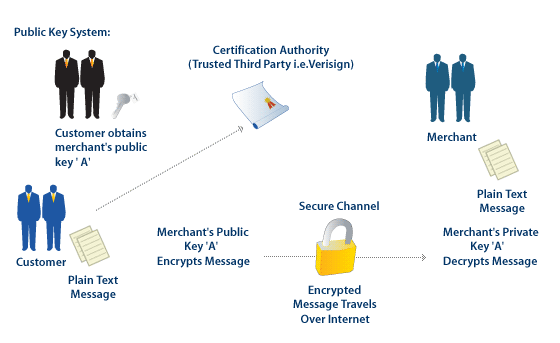Olde Theorems, Modern Applications

Fermat's Little Theorem is over 350 years old.At the time Fermat stated his little theorem (in 1640), he, let alone anyone else, imagined that it could ever be applied in a truly useful way.

Such cases stand as a testament that one should be quite wary of deeming the pursuit of any idea, even if only for its own charm and beauty, a worthless endeavor.

Thank You!

Take two large prime numbers, $q$ and $p.$
Find the product $n,$ and the totient $\varphi.$
If $e$ and $\varphi$ have GCD one
and $d$ is $e$'s inverse, then you're done!
For sending $m$ raised to the $e$
reduced mod $n$ gives secre-$c.$